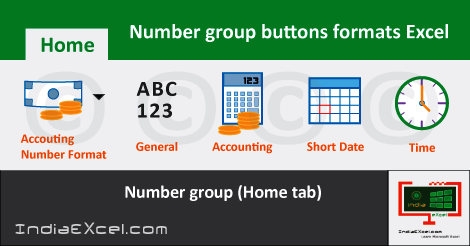# Number group tools commands Excel 2016number group in excel | number group symbols | number group names | number formats in excel

See Previous Post: Alignment Group tools buttons commands Microsoft Excel 2016

So, the fourth group is the Number Group of the Home Tab. Also, this group lies after the Clipboard, the Font & the Alignment groups in MS Excel. For instance, we could call the Number-group as the Formatting group as well.

Above all, the Format as Table of the Styles Group is also based on the formatting. The formats such as the General, the Number, the Currency and the Accounting etc; are part of the Number group.

In addition, the Accounting Number Format and the Percent Style formats are also present. Further, the Comma Style, the Increase Decimal and the Decrease Decimal formats also are available.

Some of the shortcut keys, for the formatting types are the Ctrl+Shift+~. We might pronounce this symbol “~” is the Tilde present before the 1 number key.

In addition other are the Ctrl+Shift+2Ctrl+Shift+3 etc; and so on. Particularly these shortcut keys extend till Ctrl+Shift+7 number.

Even more, the Short Date, the Long Date and the Time formats etc; also are part of the Number-group. Moreover, the rest of the formats are the Percentage, the Fraction and the Scientific formats.

Understanding what is format in Excel, is simple. So, the Format is the change in the appearance of the numbers present in cells; without any change to the original content. Lastly, below is a list of the format types present in the Number group.

## Number group buttons Microsoft Excel 2016

• General Format – Apply General format in excel.
• Number Format – Apply Number format to number in excel.
• Currency Format – Applies Currency format to number.
• Accounting Format – Apply Accounting format in excel.
• Short Date Format – Apply Short Date format to number in excel.
• Long Date Format – Applies Long Date format in excel.
• Time Format – Apply Time format to number in excel.
• Percentage Format – Applies Percentage format to number in excel.
• Accounting Number Format – Apply Accounting Number format in excel.
• Percent Style – Applies Percent format to number.
• Comma Style – Apply Comma format to number in excel.
• Increase Decimal – Used to Increase Decimal for number in excel.
• Decrease Decimal – Used to Decrease Decimal for number in excel.

See Next Post: Styles group buttons tools Home tab Microsoft Excel 2016

### Terms related to this Post:

Number Group in Excel
Number-Group in MS Excel
Number-Group Names
Number-Group-Symbols

Number-Group Excel
Number-Group Excel 2010
Number-Group-in Excel 2007
Number-Group in-Excel 2013
Number-Group in-Excel 2016